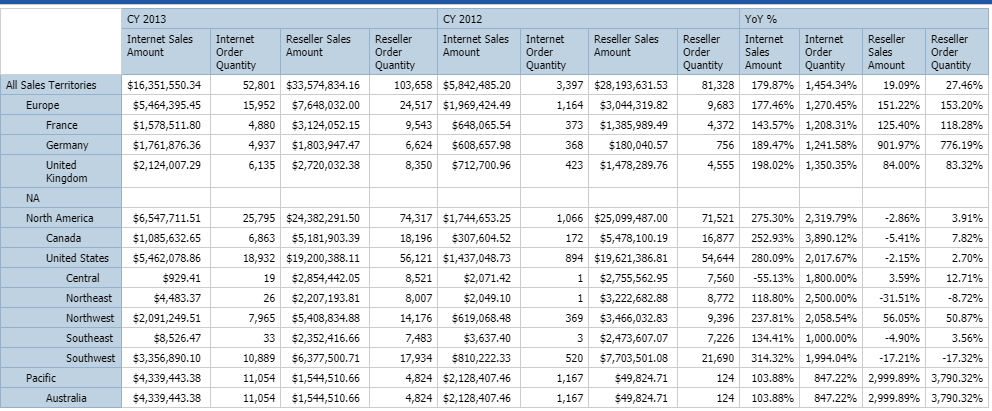# IBM Think! (And dimensional report design)

I haven’t been posting much recently, and you can always tell how busy I am by the frequency of my posts. Never fear, the crazy project I’m on will be ending soon and I can get back to posting semi-regularly.

Before anything else, a very important announcement.

I’ll be attending IBM Think! Do you want to meet me? Tell me how awesome I am and how my solutions have saved your life? Now’s your chance! Send a message to the PMsquare people here and we can set something up! Want to tell me that one of my solutions destroyed your Cognos set up? We can talk it over a beer or two in a well lit public area. During the expo, I’ll either be hanging around booth #716, or wandering around restocking my supply of branded pens and office swag.

Now to the meat of the post.

Let’s talk about dimensional report design.

Building dimensional reports is more of the more complex tasks a Cognos developer can face. Relational reports over a few tables is an easy task, but the skills learned don’t necessarily transfer over. While similar in appearance, the queries use a very different style.

When building a query, it might be tempting to explicitly create a tuple for each item. Case in point, consider the following requirement:

year in column, prior year, Year over Year%
4 measures
Org Hierarchy in rowsIt might be tempting to make each column a separate tuple.

currentyearSales: tuple([Sales],[Current Year])
priorYearSales: tuple([Sales],[Prior Year])
and so on.

But let’s take a look at the underlying MDX that’s generated.

```WITH
MEMBER [Measures].[XQE_V5M_Reseller Order Quantity YoY_CM13] AS ((([Date].[Calendar].[Calendar Year].&, [Measures].[Reseller Order Quantity])-([Date].[Calendar].[Calendar Year].&, [Measures].[Internet Order Quantity]))/([Date].[Calendar].[Calendar Year].&, [Measures].[Internet Order Quantity])), SOLVE_ORDER = 4, CAPTION = 'Reseller Order Quantity YoY'
MEMBER [Measures].[XQE_V5M_Reseller Sales Amount YoY_CM14] AS ((([Date].[Calendar].[Calendar Year].&, [Measures].[Reseller Sales Amount])-([Date].[Calendar].[Calendar Year].&, [Measures].[Reseller Sales Amount]))/([Date].[Calendar].[Calendar Year].&, [Measures].[Reseller Sales Amount])), SOLVE_ORDER = 4, CAPTION = 'Reseller Sales Amount YoY'
MEMBER [Measures].[XQE_V5M_Internet Order Quantity YoY_CM15] AS ((([Date].[Calendar].[Calendar Year].&, [Measures].[Internet Order Quantity])-([Date].[Calendar].[Calendar Year].&, [Measures].[Internet Order Quantity]))/([Date].[Calendar].[Calendar Year].&, [Measures].[Internet Order Quantity])), SOLVE_ORDER = 4, CAPTION = 'Internet Order Quantity YoY'
MEMBER [Measures].[XQE_V5M_Internet Sales Amount YoY_CM16] AS ((([Date].[Calendar].[Calendar Year].&, [Measures].[Internet Sales Amount])-([Date].[Calendar].[Calendar Year].&, [Measures].[Internet Sales Amount]))/([Date].[Calendar].[Calendar Year].&, [Measures].[Internet Sales Amount])), SOLVE_ORDER = 4, CAPTION = 'Internet Sales Amount YoY'
MEMBER [Measures].[XQE_V5M_Reseller Order Quantity 2012_CM17] AS ([Date].[Calendar].[Calendar Year].&, [Measures].[Internet Order Quantity]), SOLVE_ORDER = 4, CAPTION = 'Reseller Order Quantity 2012'
MEMBER [Measures].[XQE_V5M_Reseller Sales Amount 2012_CM18] AS ([Date].[Calendar].[Calendar Year].&, [Measures].[Reseller Sales Amount]), SOLVE_ORDER = 4, CAPTION = 'Reseller Sales Amount 2012'
MEMBER [Measures].[XQE_V5M_Internet Order Quantity 2012_CM19] AS ([Date].[Calendar].[Calendar Year].&, [Measures].[Internet Order Quantity]), SOLVE_ORDER = 4, CAPTION = 'Internet Order Quantity 2012'
MEMBER [Measures].[XQE_V5M_Internet Sales Amount 2012_CM20] AS ([Date].[Calendar].[Calendar Year].&, [Measures].[Internet Sales Amount]), SOLVE_ORDER = 4, CAPTION = 'Internet Sales Amount 2012'
MEMBER [Measures].[XQE_V5M_Reseller Order Quantity 2013_CM21] AS ([Date].[Calendar].[Calendar Year].&, [Measures].[Reseller Order Quantity]), SOLVE_ORDER = 4, CAPTION = 'Reseller Order Quantity 2013'
MEMBER [Measures].[XQE_V5M_Reseller Sales Amount 2013_CM22] AS ([Date].[Calendar].[Calendar Year].&, [Measures].[Reseller Sales Amount]), SOLVE_ORDER = 4, CAPTION = 'Reseller Sales Amount 2013'
MEMBER [Measures].[XQE_V5M_Internet Order Quantity 2013_CM23] AS ([Date].[Calendar].[Calendar Year].&, [Measures].[Internet Order Quantity]), SOLVE_ORDER = 4, CAPTION = 'Internet Order Quantity 2013'
MEMBER [Measures].[XQE_V5M_Internet Sales Amount 2013_CM12] AS ([Date].[Calendar].[Calendar Year].&, [Measures].[Internet Sales Amount]), SOLVE_ORDER = 4, CAPTION = 'Internet Sales Amount 2013'
SELECT
{CROSSJOIN({[Date].[Calendar].[Calendar Year].&}, {[Measures].[XQE_V5M_Internet Sales Amount 2013_CM12], [Measures].[XQE_V5M_Internet Order Quantity 2013_CM23], [Measures].[XQE_V5M_Reseller Sales Amount 2013_CM22], [Measures].[XQE_V5M_Reseller Order Quantity 2013_CM21]}), CROSSJOIN({[Date].[Calendar].[Calendar Year].&}, {[Measures].[XQE_V5M_Internet Sales Amount 2012_CM20], [Measures].[XQE_V5M_Internet Order Quantity 2012_CM19], [Measures].[XQE_V5M_Reseller Sales Amount 2012_CM18], [Measures].[XQE_V5M_Reseller Order Quantity 2012_CM17]}), ([Date].[Calendar].DEFAULTMEMBER, [Measures].[XQE_V5M_Internet Sales Amount YoY_CM16]), ([Date].[Calendar].DEFAULTMEMBER, [Measures].[XQE_V5M_Internet Order Quantity YoY_CM15]), ([Date].[Calendar].DEFAULTMEMBER, [Measures].[XQE_V5M_Reseller Sales Amount YoY_CM14]), ([Date].[Calendar].DEFAULTMEMBER, [Measures].[XQE_V5M_Reseller Order Quantity YoY_CM13])} DIMENSION PROPERTIES PARENT_LEVEL,  PARENT_UNIQUE_NAME ON AXIS(0),
DESCENDANTS([Sales Territory].[Sales Territory].[All Sales Territories], 3, SELF_AND_BEFORE) DIMENSION PROPERTIES PARENT_LEVEL,  PARENT_UNIQUE_NAME ON AXIS(1)
FROM [Adventure Works]  CELL PROPERTIES CELL_ORDINAL,  FORMAT_STRING,  LANGUAGE,  VALUE
```

Looking at this in the profiler, I can see that it’s taking 20 ms to process, and generates 67 subcubes.

In this case it’s better to use the years as a set, and rely on the implicit grouping of a crosstab. This results in significantly fewer data items.

But what about the Year over Year% columns? Manually calculating each column would certainly be easy to do, but again, it’s not necessary. We can create a calculated member in the time hierarchy that calculates it for us.
member(([Current Year] – [Prior Year]) / [Prior Year] , ‘YoY’,’YoY’,[Cube].[Time Dim].[Time Hier])

It might look silly to people coming from a relational background. After all, (2017-2016)/2016 = 4.96%. In this case, the calculation is happening to the nested measures. We can then select the member fact cells of the calculated member, and format all of the cells as percentage.

Let’s take a look at the underlying MDX:

WITH
MEMBER [Date].[Calendar].[XQE_V5M_CM1] AS ((([Date].[Calendar].[Calendar Year].&)-([Date].[Calendar].[Calendar Year].&))/([Date].[Calendar].[Calendar Year].&)), SOLVE_ORDER = 4, CAPTION = ‘YoY %’
SELECT
{CROSSJOIN({[Date].[Calendar].[Calendar Year].&, [Date].[Calendar].[Calendar Year].&}, {[Measures].[Internet Sales Amount], [Measures].[Internet Order Quantity], [Measures].[Reseller Sales Amount], [Measures].[Reseller Order Quantity]}), CROSSJOIN({[Date].[Calendar].[XQE_V5M_CM1]}, {[Measures].[Internet Sales Amount], [Measures].[Internet Order Quantity], [Measures].[Reseller Sales Amount], [Measures].[Reseller Order Quantity]})} DIMENSION PROPERTIES PARENT_LEVEL, PARENT_UNIQUE_NAME ON AXIS(0),
DESCENDANTS([Sales Territory].[Sales Territory].[All Sales Territories], 3, SELF_AND_BEFORE) DIMENSION PROPERTIES PARENT_LEVEL, PARENT_UNIQUE_NAME ON AXIS(1)
FROM [Adventure Works] CELL PROPERTIES CELL_ORDINAL, FORMAT_STRING, LANGUAGE, VALUE
[/sourecode]

In this case the runtime is 12 ms with 31 subcubes generated.

That’s 40% faster. While in this example the actual difference is insignificant, in real life the runtime difference for complex queries can be profound. The report that instigated this post was taking over an hour to process, but with the changes I mentioned it dropped down to 3 minutes.

The end result here is a faster, more compact query. Much easier to maintain, much easier to change, and much easier to hand off to clients so you never have to look at it again.

Report XML Below